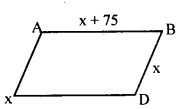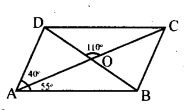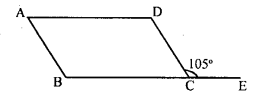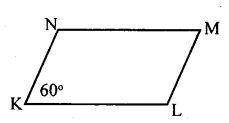# KSEEB Solutions for Class 8 Maths Chapter 15 Quadrilaterals Ex 15.3

In this chapter, we provide KSEEB SSLC Class 8 Maths Chapter 15 Quadrilaterals Ex 15.3 for English medium students, Which will very helpful for every student in their exams. Students can download the latest KSEEB SSLC Class 8 Maths Chapter 15 Quadrilaterals Ex 15.3 pdf, free KSEEB SSLC Class 8 Maths Chapter 15 Quadrilaterals Ex 15.3 pdf download. Now you will get step by step solution to each question.

### Karnataka State Syllabus Class 8 Maths Chapter 15 Quadrilaterals Ex 15.3

Question 1.
The adjacent angles of a parallelogram are in the ratio 2 : 1. Find the measures of all the angles.
The adjacent angles are in the ratio 2 : 1.
Let the angles be 2x and x
2x + x = 180°
[adjacent angles of a parallelogram are supplementary]
3x = 180° .
x =$\frac { 180 }{ 3 }$ = 60°
2x = 2 × 60° = 120°
∴The angles of the parallelogram are 60°, 120°. 60° and 120°

Question 2.
A field is in the form of a parallelogram whose perimeter is 450m and one of its sides is larger than the other by 75m. Find the lengths of all the sides.
Let one side be x, then the other side is x + 75Perimeter = 450
x + (x + 75) + x + (x + 75) = 450
4x + 150 = 450
4x = 450 – 150
4x = 300
x = 3004 = 75
x = 75m
x + 75 = 75 + 75 = 150 m
∴ The four sides are 75m, 150m, 75m, 150m.

Question 3.
In the figure ABCD is a parallelogram. The diagonals AC and BD intersect at 0; and ∠DAC = 40°, ∠CAB = 35° and ∠DOC = 110° calculate the measure ∠ABO, ∠ADC, ∠ACB and ∠CBD∠AOB = ∠DOC = 110°
[Vertically opposite angles]
In ΔAOB,
∠AOB + ∠OAB + ∠OBA = 180°
110 + 55 +∠OBA = 180°
∠OBA = 180 – 165
∠ABO = 15°
[adjacent angles of a parallelogram , are supplementary]
∠ACB = ∠DAC = 40°
∠ACB = 40°
∠ABC = ∠ ADC = 85°
[Opposite angles pf a parallelogram]
[∠ABC = ∠ABO + ∠CBO]
15 + ∠CBO = 85°
∠CBO = 85 – 15
∠CBO = 70
∴ ∠CBO = ∠CBD = 70°
∴ ∠CBD = 70°

Question 4.
In a parallelogram ABCD, the side DC is produced to E and ∠BCE = 105° calculate ∠A, ∠E, ∠C, and∠D.∠DCB + ∠DCE = 180° [Linear pair] ∠DCB + 1050 = 180°
∠DCB = 180 – 105
∠DCB = 75°
∠A = ∠DCB = 75°
∠A = ∠C = 75°
[Opposite angles of a parallelogram]
∠A +∠B = 180°
75° + ∠B = 180°
∠B = 180 + 75
∠B = 105°
∠B = ∠D = 105°
[Opposite angles of a parallelogram]

Question 5.
In a parallelogram KLMN, ∠K = 60 °. Find the measures of all the angles.∠K = ∠M = 60° [Opposite angles of parallelogram]
∠K + ∠N = 180° [adjacent angles of a parallelogram]
60 + ∠N = 180°
∠N = 180 – 60
∠N = 120°
∠N = ∠L = 120°
[Opposite angles of a parallelograom]
∴ Angles of a paralelogram are 60°, 120°, 60° & 120°

All Chapter KSEEB Solutions For Class 8 maths

—————————————————————————–

All Subject KSEEB Solutions For Class 8

*************************************************

I think you got complete solutions for this chapter. If You have any queries regarding this chapter, please comment on the below section our subject teacher will answer you. We tried our best to give complete solutions so you got good marks in your exam.

If these solutions have helped you, you can also share kseebsolutionsfor.com to your friends.

Best of Luck!!